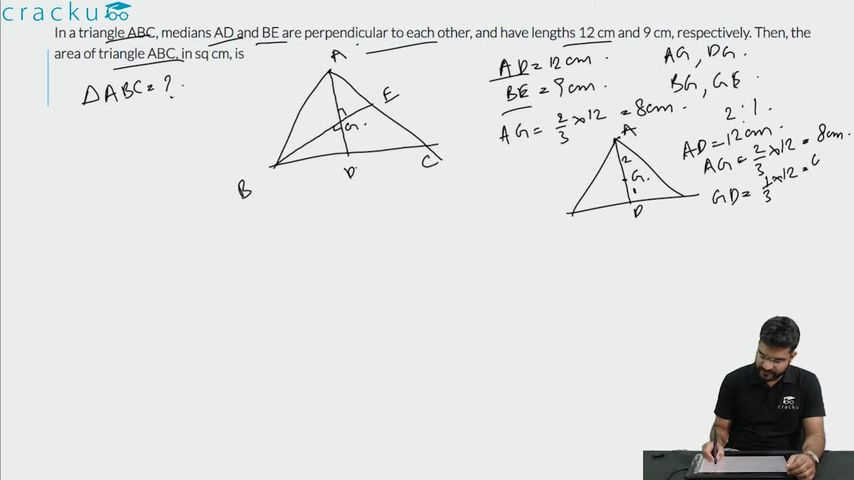Question 25

# In a triangle ABC, medians AD and BE are perpendicular to each other, and have lengths 12 cm and 9 cm, respectively. Then, the area of triangle ABC, in sq cm, is

Solution

It is given that AD and BE are medians which are perpendicular to each other.

The lengths of AD and BE are 12cm and 9cm respectively.

It is known that the centroid G divides the median in the ratio of 2:1

Area of $$\triangle$$ ABC = 2* Area of the triangle ABD

Area of $$\triangle\$$ABD = Area of $$\triangle\$$ AGB + Area of $$\triangle\$$ BGD

Since $$\angle\ AGB\ =\ \angle\ BGD\ =\ 90$$ (Given)

Area of $$\triangle\$$ AGB = $$\ \frac{\ 1}{2}\times\ 8\times\ 6$$ = 24

Area of $$\triangle\$$ BGD = $$\ \frac{\ 1}{2}\times\ 6\times\ 4$$ = 12

Area of $$\triangle\$$ABD = 24+12=36

Area of $$\triangle\ ABC\ =\ 2\times\ 36=72$$

### View Video Solution